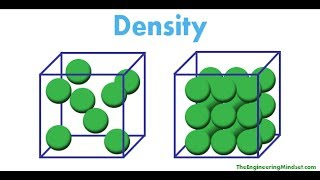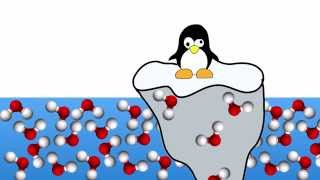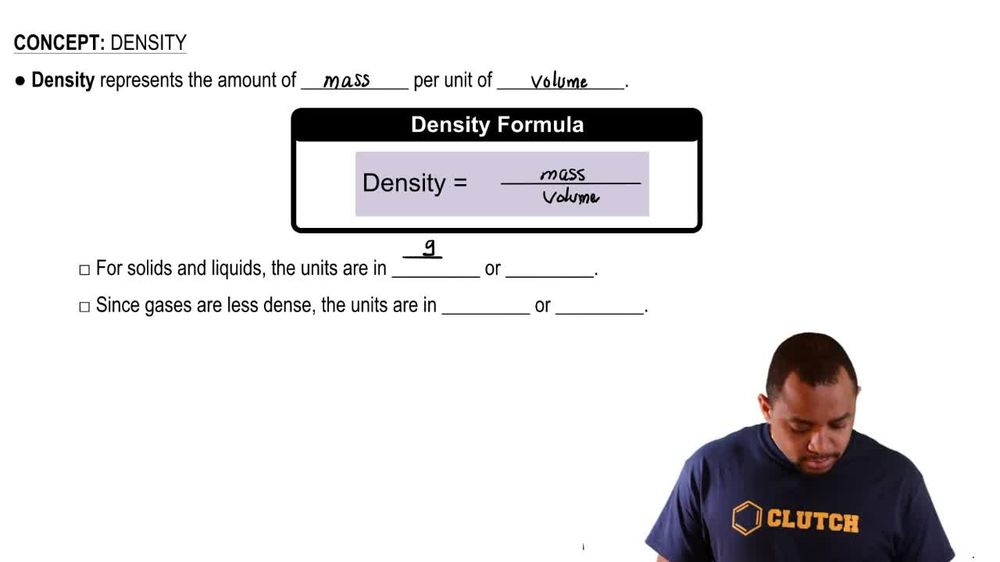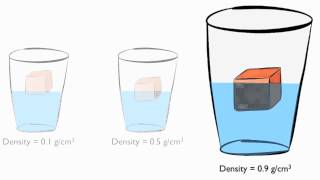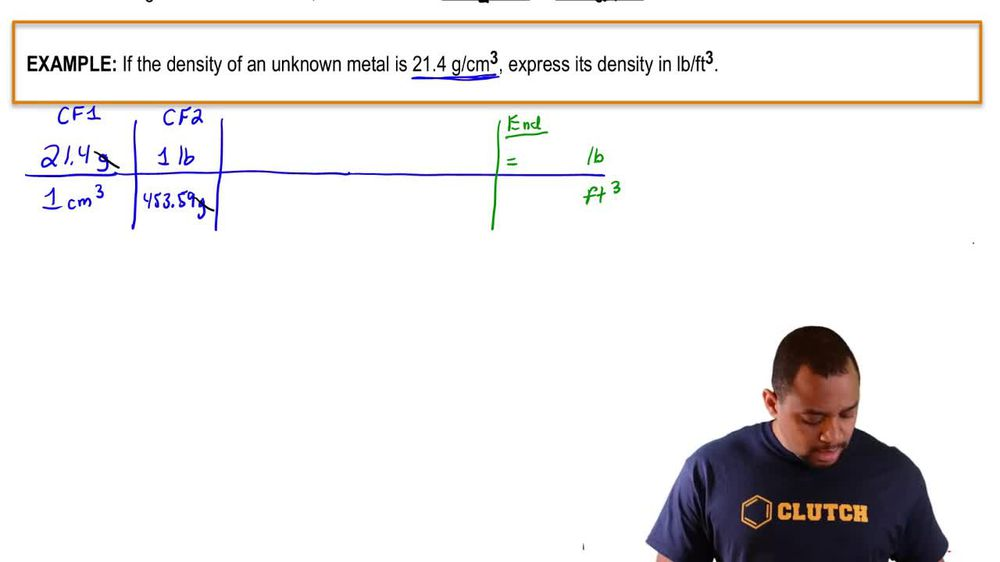Start typing, then use the up and down arrows to select an option from the list.
1. 1. Intro to General Chemistry2. Density
Problem

# More sulfuric acid (density = 1.8302 g/cm3) is produced than any other chemical—approximately 3.6 * 1011 lb/yr world-wide. What is the volume of this amount in liters?

Relevant Solution1m
Play a video:
Hey everyone. So here we're told the density of ethanol is 0.789 g per mil leader. And for part A. We need to determine the mass when given a 15.8 ml volume. And we need to express the answer in terms of 466 for B. We are given the grams of ethanol. We need to find the volume also in terms of four significant figures. Alright, so let's start out with part A. We have 15.8 mls. We take our density, which is one middle leader 10.789 g. So here mls cancel out and we'll have our grams, which comes out to 12.47 g. As our final answer For B were given 5.63 g. Because grams need to be canceled out. I need to flip my density where the grams are here on the bottom now and mls on the top here. When I work this out, that's gonna give me 7.136 mls as my final answer. So these represent the answers for both A and B respectively.